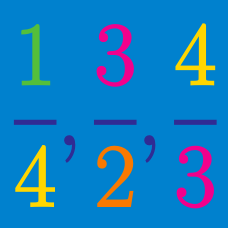Number Theory

# Converting Repeating Decimals into Fractions

Which of the following is equal to $$\frac{11}{9}$$?

A) $$1.1$$
B) $$1.\overline{1}$$
C) $$1.2$$
D) $$1.\overline{2}$$
E) $$1.9$$

Details and assumptions:

$$9.\overline{3} = 9.33333...$$

\begin{align*} 0.111111\ldots &= \frac{1}{9} \\ \\ 0.121212\ldots &= \ ? \end{align*}

Express $$1 . 2 \overline{94}$$ as a fraction.

Evaluate $$\displaystyle{\frac{ 0 . \overline{26} }{ 9 . \overline{7} }}$$ by changing the decimals into fractions.

Which of the following is equal to $$0.\overline{21}$$?

Details and assumptions:

$$9.\overline{3} = 9.33333...$$

×